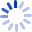# Applied Linear Statistical Models

by

Focusing on applied statistical models, this text has an applied approach with an emphasis on understanding of concepts and exposition by means of examples. Theoretical foundations are provided so that applications of regression analysis can be carried out. There is expanded use of graphics, scatter plot metrics, and 3D rotating plots. Case studies feature throughout the text.

##### Cash for Textbooks